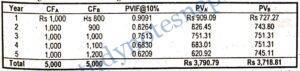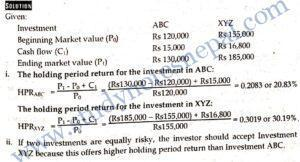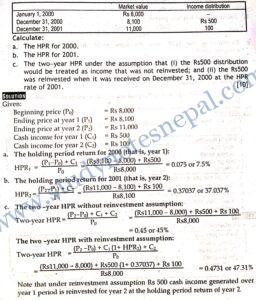###### 1. Differentiate between expected return and required return?

The expected return refers to the rate of return expected from an investment. On the other hand required rate of return is the rate of return that an investment must earn to compensate against the level of risk involved in it. Expected return and required returns are two important measures of returns in investment decision. The required return is the minimum return that an investment must produce. Any investment is considered worthwhile only if the expected rate of return from the investment is greater than the required rate of return.”

###### 2. What do you understand by a risk-averse investor?

A risk-averse investor is one who does not prefer risk. A risk-averse investor always prefers maximum return and minimum risk. Given the same level of returns from two investment alternatives, a risk-averse investor always prefers the one with lower risk. Putting it in other way, given the same level of risk of two alternatives, the risk-averse investor prefers one with the highest return. In investment theory, it is assumed that all investors are risk-averse. However. the degree of risk aversion varies from one investor to other. A highly risk averse investor prefers low risk-low return alternative while a less risk-averse investor prefers high risk-high return alternative.

###### 3. Consider two investment alternatives: Stock X with 10 percent expected return and 20 percent standard deviation; and Stock Y with 15 percent expected return and 25 percent standard deviation. Which investment is better in terms of risk per unit of return?

SOLUTION

Given:

Expected return on Stock X, (E(Rx) = 10%

Standard deviation of Stock X x = 20%

Expected return on Stock Y, (E(Ry) = 15%

Standard deviation of Stock X (Y) = 25%

Calculation of coefficient of variation:

CV =  /E(R₁)

For Stock X:

CVX: = 20  /10=2

For Stock Y:

CVY = 25 / 15 = 1.67

Investment Y is better because it has lower risk per unit of return.

###### 4. If you invest Rs 100,000 in an investment vehicle that will pay you back Rs 180,000 in seven years, what will be the yield on this investment?

SOLUTION

Given:

Present value (PV) = Rs 100,000

Future value (FVn) = Rs 180,000

Yield = (FVn / PV)^ 1/n = 180,000^ 1/7 – 1

= 0.0876 or 8.76%

Hence, the yield on this investment is 8.76 percent.

###### 5. Given a risk-free rate of 7 percent and expected inflation premium 3 percent, what is the real rate of return? What does it mean?

SOLUTION

Given:

Risk-free rate (RF) = 7%

Expected inflation premium (IP) = 3%

The real rate of return is given by:

k* = RF – IP = 7% -3% = 4%

The real risk-free rate of return is 4 percent. It is the real growth in the value of investment in risk-free assets after taking into account the impact of inflation.

###### 1. Explain the different types of risk associated with investing in bond. What is the most important source of risk for bonds in general?

Various types of risks are associated with investing in bond, depending on the nature and issue character of bonds. Some of the important risks factors that. prospective investors should consider prior to making a decision to invest in bonds are as follows:

• Interest rate risk: Rising interest rates are a key risk for bond investors. Generally, rising interest rates will result in falling bond prices. It reflects the ability of investors to obtain an attractive rate of interest on their money elsewhere. Conversely, falling interest rates will result in rising bond prices, and falling yields. Before investing in bonds, the investor should assess a bond’s duration in conjunction with the outlook for interest rates. It enables them to ensure that they are comfortable with the potential price volatility of the bond resulting from interest rate fluctuations.
• Credit risk: Credit risk is the risk that an issuer of bond will be unable to make interest or principal payments when they are due. Bond rating agencies such as Moody’s and Standard & Poor’s assess the creditworthiness of issuers and assign a credit rating based on their ability to repay obligations. Bond investors should examine the ratings of an issuer in order to establish the credit risk of a bond. High quality rating bonds are considered having lower credit risk and low quality rating are considered to be more likely to default, and thus are considered more speculative and subject to more price volatility.
• Inflation risk: Inflation reduces the purchasing power of a bond’s future coupons and principal. Bonds do not offer extraordinarily high returns, and therefore they are exposed to risk when inflation rises. Inflation leads to higher market interest rates, which affects bond prices adversely. However, there are also the adjustable rate bonds that are structured to protect investors from the risk of inflation. In such bonds, the coupon payments increase in line with the rate of inflation.
• Reinvestment risk: Bonds are also exposed to reinvestment risk particularly when market interest rate declines. When interest rates decline, investors may I have to reinvest their coupon income and their principal at maturity at lower prevailing rates. This is called a reinvestment risk.
• Liquidity risk: Liquidity risk is associated with marketability of bonds. Investors have to face liquidity risk when they are in difficulty to find a buyer and forced to sell bonds at significant discounted price when they want to sell the bonds. Bonds tend to be most liquid in the period immediately after issue. iquidity risk is usually lower for government bonds than for corporate bonds. is is because of the extremely large issue sizes of most government bonds.

These  are just some of the risks that are associated with an investment in bonds. Among these sources of risk, interest rate risk, reinvestment risk and credit risk are most common source of risk Market interest rates keep on changing. Therefore, interest rate risk and reinvestment risk are important for bond investment as bond usually offers a fixed coupon. Besides, issuers characteristics such as change in financial condition of the issuer exposes the bonds to credit risk more frequently.

###### 2. What are the factors affecting investment returns? Explain.

Investment returns are affected by many factors. These factors are classified as internal and external factors. They are described below:

• Internal factors: Internal factors refer to the factors associated with securities issuer. They consist of a set of factors that are under the control of firm issuing securities. The type of securities, management efficiency, and nature of financing used by issuing firms are some examples of internal factors affecting investment returns. For example, the investment returns depend on the types of securities such as stocks and bonds. The level of returns expected from stock investment is always higher than bond investment primarily due to higher risk exposure of stock investment. Similarly, if a firm’s management is efficient, the rate of return expected from investment in such firm is higher.
• External factors: External factors consist of a set of factors that affect not only an individual firm but whole industry and economy. These factors are uncontrollable for an individual firm. General business condition, inflation, monetary and fiscal policy of government, change in foreign exchange rate, political instability in the country, etc. are some external factors affecting investment returns. For example, in the conducive political environment, there are productive investment opportunities so we expect higher returns from investment in such environment. Similarly, level of inflation also affects the investment return. In an inflationary environment, value of real estate investment grows up while the value of fixed income securities is adversely affected.
###### 3. What is the role of the concept of time value of money in selecting investment alternatives? Which investment do you prefer according to this concept? Explain.

The time value of money is the concept used to understand value of cash flows occurring over different time period. According to this concept, every present sum of money is more valuable than the equal future some of money. This happens simply because the present sum of money potentially earns additional sum of money in future. Thus, according to the concept of time value of money, the investment that produces larger cash flows in earlier period is more valuable than the investment that produces the equal larger cash flows in the later period. For example, let us consider two investments: Investment A and B. Suppose both investments require equal investment of Rs 1,000 each at the beginning and both offer 10 percent return. Thus, total annual return from the Investment A is Rs 100 and it is also Rs 100 from Investment B. However, assume that Investment A pays Rs 100 return all at the end of year while Investment B pays Rs 50 six months. In this situation, Investment B is more valuable than Investment A because each Rs 50 in return at the end of six month period can be reinvested for the next six month. Investment B is valuable simply because its gives earlier cash flows than Investment A. Thus time value of money is important in selecting investment alternatives.

The time value of money concept does not only consider the timing of returns but also takes into account the level of risk associated with the investment. Under time value money concept, we use present value and future value techniques to decide on the investment alternatives. The investment alternative that has larger present value or larger future value of cash flows is considered better if all other things remain the same. To illustrate, let us consider the present value of cash flows of Security A and B discounted at 10 percent rate or return as given in the following Table.We assume that both securities are in equal risk class so we have selected the same discount rate to work-out present value of cash flows. Over five years of investment period both securities produce an equal total cash flows of Rs 5,000. However, the present value of cash flows associated with security A is higher than that of security B. If we assume that the level of investment required at the beginning is equal for both security, we select the security A with higher present value.

###### 3. Explain basic types of risk preference of investors. Which of the risk behaviour is the most common to investors? Explain.

Different risk class investment alternatives are available. Some alternatives have higher risk and also offer higher return, while other alternatives have lower risk and offer lower return. Investors generally prefer lower risk to higher risk. Selection of an appropriate investment vehicle is based on the individual’s preference towards risk. Before selecting among the investment alternatives, investors consider their preference toward risk. From an individual’s point of view, there are three types of risk preference of investors: risk indifferent, risk-averse and risk seeker. They are described below.

• Risk indifferent: A risk indifferent investor is neutral toward risk preference. The required return is invariant for such investor for any level of risk.
• Risk-averse: A risk-averse investor is one who prefers higher return to lower return, and lower risk to higher risk. For a given level of investment risk, a risk-averse investor always seeks to invest in alternatives offering higher return. In other words, a risk-averse investor prefers low-risk investment for a given level of return.
• Risk-seeker: A risk-seeker investor enjoys taking risk. S/he prefers higher risk and, is ready to sacrifice some part of the returns for enjoying the adventure of high-risk investment.

Theories and observations show that risk-averse behavior is most common to investors. Investors are risk-averse primarily due to the diminishing marginal utility of wealth. However, the degree of risk-aversion varies from one investor to other. Some investors are highly risk-averse and prefer low risk low return investments. Such investors, for example, tend to invest in bonds rather than common stock. On the other hand, some investors are less risk averse, and they prefer high risk- high return investment. Such investors prefer to invest in common stocks rather than bonds.

1. You purchased 1000 shares of Kathmandu Finance Company at Rs 150 per share and 500 shares of NABIL at Rs 650 per share in Jan 10, 2003 Kathmandu Finance did not declared any dividend this year but paid cash dividend of Rs 10 per share in 2004, while NABIL declared and paid dividend of Rs 50 and 60 per share in 2003 and 2004. You sold the stock of Kathmandu Finance at Rs 160 per share and the shares of NABIL at Rs 825 per share after a two year holding period.

1. Calculate the two-year holding period return on your total investment, assuming that the dividend in 2003 was not reinvested.
2. Calculate the two-year holding period, assuming that the dividend received in 2003 was reinvested at 10 percent.

SOLUTION###### 2.Information are given below3. Mr. Thapa, a financial analyst for Gandaki Noodles Pvt. Ltd., wishes to estimate the rate of return for two similar risk investments- ABC and XYZ. Thapa’s research indicates that the immediate past returns will act as reasonable estimates of future returns. A year earlier, investment ABC had a market value of Rs 120,000 and investment XYZ, of Rs 155,000. During the year, investment ABC generated cash flow of Rs 15,000 and investment XYZ generated cash flow of Rs 16,800. The current market values of investments ABC and XYZ are Rs 130,000 and Rs 185,000 respectively.

1. Calculate the expected holding period rate of return on investment ABC and XYZ using the most recent year’s data.
2. If the two investments are equally risky, which one should Mr. Thapa recommend? Why?###### 4. Suppose that you have the following data on the performance of your tax-deferred portfolio: